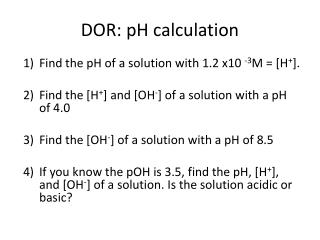DownloadDownload PresentationDOR: pH calculation

# DOR: pH calculation

Télécharger la présentation## DOR: pH calculation

- - - - - - - - - - - - - - - - - - - - - - - - - - - E N D - - - - - - - - - - - - - - - - - - - - - - - - - - -
##### Presentation Transcript

1. DOR: pH calculation • Find the pH of a solution with 1.2 x10 -3M = [H+]. • Find the [H+] and [OH-] of a solution with a pH of 4.0 • Find the [OH-] of a solution with a pH of 8.5 • If you know the pOH is 3.5, find the pH, [H+], and [OH-] of a solution. Is the solution acidic or basic?

2. DOR: pH State whether the following solutions are basic, acidic, or neutral. • pH = 3.0 • pH = 7.6 • pH = 11.3 • pH = 2.5 • pH = 7.0

3. DOR: pH calculation 4/12 • Find the pH of a solution with 1 x10 -3M = [H+] • Find the pH of a solution with 1 x 10 -4M = [H+] • Find the [H+] and [OH-] of a solution with a pH of 8. • If you know the pOH is 3.5, find the pH. Is the solution acidic or basic? 1) pH =3 2) pH = 11 3) pH = 9 4) pH= 1

4. Acid/Base TitrationBuffers

5. Titration • Used to determine UNKNOWN concentrations of solutions through a solution of KNOWN concentration. • An acidic or basic solution of KNOWN concentration is added to an acidic/basic solution of UNKNOWN concentration. • Indicators such as phenolphtalein display a change when all of the acid or base has been neutralized.

6. Titration Terminology • End Point: point in a titration where a change is observed due to indicator. • Equivalence Point: point in a titration where enough base or acid is present to neutralize the acidic or basic solution. • We can plot a titration on a graph—titration curve

7. Example 1: • We have two solutions (H2SO4 and KOH). The KOH solution has a concentration of 0.1500M and takes 42.61ml to titrate 25.0ml of the sulfuric acid solution. What is the concentration of the H2SO4solution?

8. DOR: pH calculation 2 4/12 State whether a solution is acidic, basic or neutral. • pH = 5 • [H+] = 1 x 10-11 M • pOH = 12 Find the following: 4) If a solution has a pH of 3.25, what is the [H+] and [OH-] ? Is the solution acidic, basic, or neutral?

9. Example 2 • 14.5ml of HNO3 was neutralized in a titration using 12.5ml of Ba(OH)2 with a 1.75x10-2M concentration. What is the concentration of the HNO3 solution?

10. Strength of Acids and Bases • Based on the concentration of H+ or OH- ions in a solution. • Strong Acids/Bases: completely dissociate into ions in a solution. • Weak Acids/Bases: do NOT completely dissociate into ions in a solution.

11. HNO3 + NH3 NH4+ + NO3- • Identify the Acid and Base

12. Stronger the acid, weaker its conjugate base. • Stronger the base, weaker its conjugate acid.

13. Neutralization ACID + BASE SALT + WATER

14. Buffers • A mixture composed of a weak acid and its conjugate base (acidic buffer) OR weak base and its conjugate acid (basic buffer) • Allow small amounts of acids and bases into a solution without a huge change in the solution’s pH • Buffer capacity—trait of buffers indicating how much strong acid/base can be tolerated before a solution’s pH changes drastically.

15. Why are buffers important to us? • Buffers present in our body keep fluids within a certain pH range. • Blood has a pH range of approximately 7.3-7.4. • A buffer involving H2CO3/HCO3- maintains the blood pH